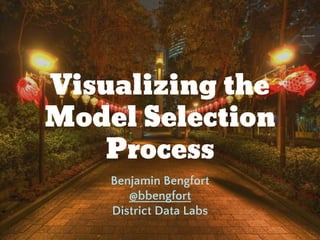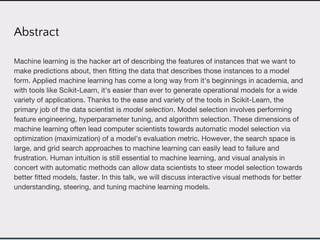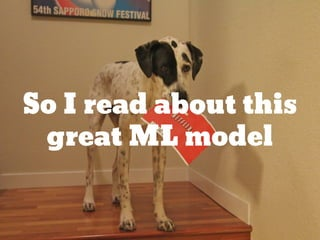Successfully reported this slideshow.

# Visualizing the Model Selection Process×

1 of 59 Ad
1 of 59 Ad

# Visualizing the Model Selection Process

Machine learning is the hacker art of describing the features of instances that we want to make predictions about, then fitting the data that describes those instances to a model form. Applied machine learning has come a long way from it's beginnings in academia, and with tools like Scikit-Learn, it's easier than ever to generate operational models for a wide variety of applications. Thanks to the ease and variety of the tools in Scikit-Learn, the primary job of the data scientist is model selection. Model selection involves performing feature engineering, hyperparameter tuning, and algorithm selection. These dimensions of machine learning often lead computer scientists towards automatic model selection via optimization (maximization) of a model's evaluation metric. However, the search space is large, and grid search approaches to machine learning can easily lead to failure and frustration. Human intuition is still essential to machine learning, and visual analysis in concert with automatic methods can allow data scientists to steer model selection towards better fitted models, faster. In this talk, we will discuss interactive visual methods for better understanding, steering, and tuning machine learning models.

Machine learning is the hacker art of describing the features of instances that we want to make predictions about, then fitting the data that describes those instances to a model form. Applied machine learning has come a long way from it's beginnings in academia, and with tools like Scikit-Learn, it's easier than ever to generate operational models for a wide variety of applications. Thanks to the ease and variety of the tools in Scikit-Learn, the primary job of the data scientist is model selection. Model selection involves performing feature engineering, hyperparameter tuning, and algorithm selection. These dimensions of machine learning often lead computer scientists towards automatic model selection via optimization (maximization) of a model's evaluation metric. However, the search space is large, and grid search approaches to machine learning can easily lead to failure and frustration. Human intuition is still essential to machine learning, and visual analysis in concert with automatic methods can allow data scientists to steer model selection towards better fitted models, faster. In this talk, we will discuss interactive visual methods for better understanding, steering, and tuning machine learning models.

## More Related Content

### Visualizing the Model Selection Process

1. 1. Visualizing the Model Selection Process Benjamin Bengfort @bbengfort District Data Labs
2. 2. Abstract Machine learning is the hacker art of describing the features of instances that we want to make predictions about, then fitting the data that describes those instances to a model form. Applied machine learning has come a long way from it's beginnings in academia, and with tools like Scikit-Learn, it's easier than ever to generate operational models for a wide variety of applications. Thanks to the ease and variety of the tools in Scikit-Learn, the primary job of the data scientist is model selection. Model selection involves performing feature engineering, hyperparameter tuning, and algorithm selection. These dimensions of machine learning often lead computer scientists towards automatic model selection via optimization (maximization) of a model's evaluation metric. However, the search space is large, and grid search approaches to machine learning can easily lead to failure and frustration. Human intuition is still essential to machine learning, and visual analysis in concert with automatic methods can allow data scientists to steer model selection towards better fitted models, faster. In this talk, we will discuss interactive visual methods for better understanding, steering, and tuning machine learning models.
4. 4. Koren, Yehuda, Robert Bell, and Chris Volinsky. "Matrix factorization techniques for recommender systems." Computer 42.8 (2009): 30-37.
5. 5. def nnmf(R, k=2, steps=5000, alpha=0.0002, beta=0.02): n, m = R.shape P = np.random.rand(n,k) Q = np.random.rand(m,k).T for step in range(steps): for idx in range(n): for jdx in range(m): if R[idx][jdx] > 0: eij = R[idx][jdx] - np.dot(P[idx,:], Q[:,jdx]) for kdx in range(K): P[idx][kdx] = P[idx][kdx] + alpha * (2 * eij * Q[kdx][jdx] - beta * P[idx][kdx]) Q[kdx][jdx] = Q[kdx][jdx] + alpha * (2 * eij * P[idx][kdx] - beta * Q[kdx][jdx]) e = 0 for idx in range(n): for jdx in range(m): if R[idx][jdx] > 0: e += (R[idx][jdx] - np.dot(P[idx,:], Q[:,jdx])) ** 2 if e < 0.001: break return P, Q.T
6. 6. Life with Scikit-Learn
7. 7. from sklearn.decomposition import NMF model = NMF(n_components=2, init='random', random_state=0) model.fit(R)
8. 8. from sklearn.decomposition import NMF, TruncatedSVD, PCA models = [ NMF(n_components=2, init='random', random_state=0), TruncatedSVD(n_components=2), PCA(n_components=2), ] for model in models: model.fit(R)
9. 9. So now I’m all
10. 10. Made Possible by the Scikit-Learn API Buitinck, Lars, et al. "API design for machine learning software: experiences from the scikit-learn project." arXiv preprint arXiv:1309.0238 (2013). class Estimator(object): def fit(self, X, y=None): """ Fits estimator to data. """ # set state of self return self def predict(self, X): """ Predict response of X """ # compute predictions pred return pred class Transformer(Estimator): def transform(self, X): """ Transforms the input data. """ # transform X to X_prime return X_prime class Pipeline(Transfomer): @property def named_steps(self): """ Returns a sequence of estimators """ return self.steps @property def _final_estimator(self): """ Terminating estimator """ return self.steps[-1]
11. 11. Algorithm design stays in the hands of Academia
12. 12. Wizardry When Applied
13. 13. The Model Selection Triple Arun Kumar http://bit.ly/2abVNrI Feature Analysis Algorithm Selection Hyperparameter Tuning
14. 14. The Model Selection Triple - Define a bounded, high dimensional feature space that can be effectively modeled. - Transform and manipulate the space to make modeling easier. - Extract a feature representation of each instance in the space. Feature Analysis
15. 15. Algorithm Selection The Model Selection Triple - Select a model family that best/correctly defines the relationship between the variables of interest. - Define a model form that specifies exactly how features interact to make a prediction. - Train a fitted model by optimizing internal parameters to the data.
16. 16. Hyperparameter Tuning The Model Selection Triple - Evaluate how the model form is interacting with the feature space. - Identify hyperparameters (parameters that affect training or the prior, not prediction) - Tune the fitting and prediction process by modifying these params.
17. 17. Can it be automated?
18. 18. Regularization is a form of automatic feature analysis. X0 X1 X0 X1 L1 Normalization Possibility that a feature is eliminated by setting its coefficient equal to zero. L2 Normalization Features are kept balanced by minimizing the relative change of coefficients during learning.
19. 19. Automatic Model Selection Criteria from sklearn.cross_validation import KFold kfolds = KFold(n=len(X), n_folds=12) scores = [ model.fit( X[train], y[train] ).score( X[test], y[test] ) for train, test in kfolds ] F1 R2
20. 20. Automatic Model Selection: Try Them All! from sklearn.svm import SVC from sklearn.neighbors import KNeighborsClassifier from sklearn.ensemble import RandomForestClassifier from sklearn.ensemble import AdaBoostClassifier from sklearn.naive_bayes import GaussianNB from sklearn import cross_validation as cv classifiers = [ KNeighborsClassifier(5), SVC(kernel="linear", C=0.025), RandomForestClassifier(max_depth=5), AdaBoostClassifier(), GaussianNB(), ] kfold = cv.KFold(len(X), n_folds=12) max([ cv.cross_val_score(model, X, y, cv=kfold).mean for model in classifiers ])
21. 21. Automatic Model Selection: Search Param Space from sklearn.feature_extraction.text import * from sklearn.linear_model import SGDClassifier from sklearn.grid_search import GridSearchCV from sklearn.pipeline import Pipeline pipeline = Pipeline([ ('vect', CountVectorizer()), ('tfidf', TfidfTransformer()), ('model', SGDClassifier()), ]) parameters = { 'vect__max_df': (0.5, 0.75, 1.0), 'vect__max_features': (None, 5000, 10000), 'tfidf__use_idf': (True, False), 'tfidf__norm': ('l1', 'l2'), 'model__alpha': (0.00001, 0.000001), 'model__penalty': ('l2', 'elasticnet'), } search = GridSearchCV(pipeline, parameters) search.fit(X, y)
22. 22. Maybe not so Wizard?
23. 23. Automatic Model Selection: Search? Search is difficult particularly in high dimensional space. Even with techniques like genetic algorithms or particle swarm optimization, there is no guarantee of a solution. As the search space gets larger, the amount of time increases exponentially.
24. 24. Anscombe, Francis J. "Graphs in statistical analysis." The American Statistician 27.1 (1973): 17-21. Anscombe’s Quartet
25. 25. Through visualization we can steer the model selection process
26. 26. Model Selection Management Systems Kumar, Arun, et al. "Model selection management systems: The next frontier of advanced analytics." ACM SIGMOD Record 44.4 (2016): 17-22. Optimized Implementations User Interfaces and DSLs Model Selection Triples { {FE} x {AS} X {HT} }
27. 27. Can we visualize machine learning?
28. 28. Data Management Wrangling Standardization Normalization Selection & Joins Model Evaluation + Hyperparameter Tuning Model Selection Feature Analysis Linear Models Nearest Neighbors SVM Ensemble Trees Bayes Feature Analysis Feature Selection Model Selection Revisit Features Iterate! Initial Model Model Storage
29. 29. Data and Model Management
30. 30. Is “GitHub for Data” Enough?
31. 31. Visualizing Feature Analysis
32. 32. SPLOM (Scatterplot Matrices)
33. 33. Seo, Jinwook, and Ben Shneiderman. "A rank-by-feature framework for interactive exploration of multidimensional data." Information visualization 4.2 (2005): 96-113. Visual Rank by Feature: 1 Dimension Rank by: 1. Normality of distribution (Shapiro-Wilk and Kolmogorov-Smirnov) 2. Uniformity of distribution (entropy) 3. Number of potential outliers 4. Number of hapaxes 5. Size of gap
34. 34. Seo, Jinwook, and Ben Shneiderman. "A rank-by-feature framework for interactive exploration of multidimensional data." Information visualization 4.2 (2005): 96-113. Visual Rank by Feature: 1 Dimension Rank by: 1. Normality of distribution (Shapiro-Wilk and Kolmogorov-Smirnov) 2. Uniformity of distribution (entropy) 3. Number of potential outliers 4. Number of hapaxes 5. Size of gap
35. 35. Seo, Jinwook, and Ben Shneiderman. "A rank-by-feature framework for interactive exploration of multidimensional data." Information visualization 4.2 (2005): 96-113. Visual Rank by Feature: 2 Dimensions Rank by: 1. Correlation Coefficient (Pearson, Spearman) 2. Least-squares error 3. Quadracity 4. Density based outlier detection. 5. Uniformity (entropy of grids) 6. Number of items in the most dense region of the plot.
36. 36. Joint Plots: Diving Deeper after Rank by Feature Special thanks to Seaborn for doing statistical visualization right!
37. 37. Detecting Separablity
39. 39. Parallel Coordinates
40. 40. Decomposition (PCA, SVD) of Feature Space
41. 41. Visualizing Model Selection
42. 42. Confusion Matrices
43. 43. Receiver Operator Characteristic (ROC) and Area Under Curve (AUC)
44. 44. Prediction Error Plots
45. 45. Visualizing Residuals
46. 46. Model Families vs. Model Forms vs. Fitted Models Rebecca Bilbro http://bit.ly/2a1YoTs
47. 47. kNN Tuning Slider in 2 Dimensions Scott Fortmann-Roe http://bit.ly/29P4SS1
48. 48. Visualizing Evaluation/Tuning
49. 49. Cross Validation Curves
50. 50. Visual Grid Search
51. 51. Integrating Visual Model Selection with Scikit-Learn Yellowbrick
52. 52. Scikit-Learn Pipelines: fit() and predict() Data Loader Transformer Transformer Estimator Data Loader Transformer Transformer Estimator Transformer
53. 53. Yellowbrick Visual Transformers Data Loader Transformer(s) Feature Visualization Estimator fit() draw() predict() Data Loader Transformer(s) EstimatorCV Evaluation Visualization fit() predict() score() draw()
54. 54. Model Selection Pipelines Multi-Estimator Visualization Data Loader Transformer(s) EstimatorEstimatorEstimatorEstimator Cross Validation Cross Validation Cross Validation Cross Validation
55. 55. Employ Interactivity to Visualize More Health and Wealth of Nations Recreated by Mike Bostock Originally by Hans Rosling http://bit.ly/29RYBJD
56. 56. Visual Analytics Mantra: Overview First; Zoom & Filter; Details on Demand Heer, Jeffrey, and Ben Shneiderman. "Interactive dynamics for visual analysis." Queue 10.2 (2012): 30.
57. 57. Codename Trinket Visual Model Management System
58. 58. Yellowbrick http://bit.ly/2a5otxB DDL Trinket http://bit.ly/2a2Y0jy DDL Open Source Projects on GitHub
59. 59. Questions!